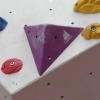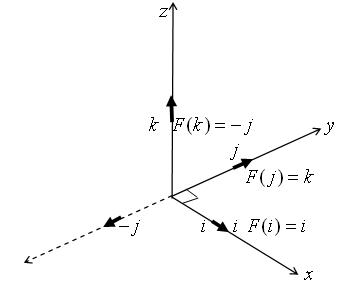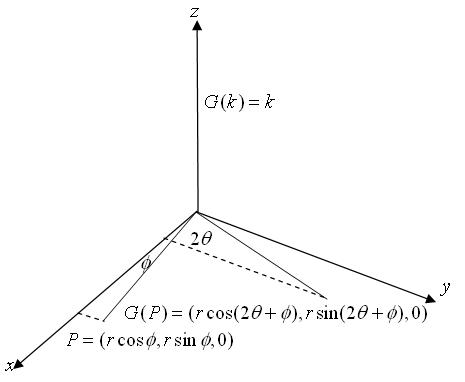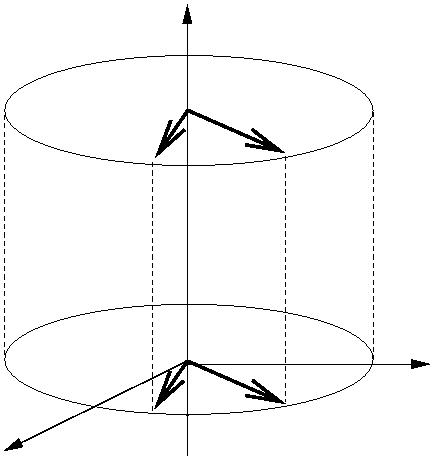#### You may also likeA quadrilateral changes shape with the edge lengths constant. Show the scalar product of the diagonals is constant. If the diagonals are perpendicular in one position are they always perpendicular?### Three by One

There are many different methods to solve this geometrical problem - how many can you find?### Tetra Perp

Show that the edges AD and BC of a tetrahedron ABCD are mutually perpendicular when: AB²+CD² = AC²+BD².

# Quaternions and Rotations

##### Age 16 to 18 Challenge Level:

Andrei from Romania sent in a good solution to this problem.

(1)(a) We have to show that $qq^{-1} = 1$: $$q^{-1} = ({1\over \sqrt 2} + {1\over \sqrt 2}{\bf i}) ({1\over \sqrt 2} - {1\over \sqrt 2}{\bf i}) = {1\over 2}(1 - {\bf i}^2) = 1$$ (b)Take $x = ti$ to be any point on the x-axis. Then $qx = ({1\over \sqrt 2} + {1\over \sqrt 2}{\bf i})t{\bf i} = {-1\over \sqrt 2} + {1\over \sqrt 2}{\bf i})t = xq.$

We have shown that $qx = xq$ and so $qxq^{-1} = x$.Let $F(v) = qvq^{-1}$ be a mapping of points $v$ in $R^3$ to images in $R^3$ where the 4-dimensional quaternion $q$ acts as an operator. We have proved that this mapping fixes every point on the x axis.

(c)What effect does this mapping have on other points in $R^3$? $$F({\bf j})= qjq^{-1} = ({1\over \sqrt 2} + {1\over \sqrt 2}{\bf i})({\bf j}) ({1\over \sqrt 2} - {1\over \sqrt 2}{\bf i})$$ $$= {1\over 2}(1+{\bf i})({\bf j})((1 - {\bf i})$$ $$= {1\over 2}({\bf j} + {\bf k})(1 - {\bf i})$$ $$= {1\over 2}({\bf j} + {\bf k} + {\bf k} - {\bf j})$$ $$= {\bf k}.$$ Similarly $F{\bf k} = -{\bf j}$. Parts (b) and (c) together show that the mapping $F(v) = qvq^{-1}$, where $q = \cos (\pi /4) + \sin (\pi /4) {\bf i}$, gives a rotation of $\pi /2$ about the x axis.(2) In this section we consider the mapping $G(v) = qvq^{-1}$ of $R^3$ to $R^3$ where the quaternion $q = \cos \theta + \sin \theta {\bf k}$ is an operator.

(a) $(cos \theta + \sin\theta k)(\cos \theta - \sin \theta k) = \cos^2 \theta + \sin^2 \theta = 1$ so these two quaternions are multiplicative inverses.

(b) We have $qk = -\sin \theta + \cos \theta k = kq$ and hence $qkq^{-1} = k$. So the mapping $G(v) = qvq^{-1}$ fixes the z-axis.

(c) What effect does the mapping $G$ have on vectors in $R^3$? We consider the vector $v = r(\cos \phi {\bf i} + \sin \phi {\bf j})$. $$qvq^{-1} =(\cos \theta + \sin \theta {\bf k})r(\cos \phi {\bf i} + \sin \phi {\bf j}) (\cos \theta - \sin \theta {\bf k})$$ $$= r((\cos \theta\cos \phi - \sin \theta \sin \phi){\bf i} +(\cos \theta \sin \phi + \sin \theta \cos \phi){\bf j}) \cos \theta {\bf i} -\sin \theta {\bf k})$$ $$= r(\cos (\theta + \phi) {\bf i} + \sin (\theta + \phi){\bf j}) (\cos \theta {\bf i} -\sin \theta {\bf k})$$ $$= r((\cos (\theta +\phi)\cos \theta - \sin (\theta + \phi)\sin \phi){\bf i} + (\cos (\theta +\phi)\sin \theta + \sin (\theta + \phi)\cos phi){\bf j})$$ $$= r(\cos (2\theta + \phi){\bf i} + \sin (2\theta + \phi){\bf j}).$$We have shown $qkq^{-1} = k$ so $G(v+tk)= q(v +tk)q^{-1} = qvq^{-1} + qtkq^{-1} = qvq^{-1} + tk$ for all $t$. We can see that the vector $v$ in the $xy$ plane is rotated about the $z$ axis by an angle $2\theta$ and all points on the vertical line through it are also rotated about the $z$-axis by an angle $2\theta$. So by the mapping $G(v) = qvq^{-1}$ all points in $R^3$ are rotated by $2\theta$ about the $z$-axis. Note that, for any rotation of $R^3$, we can make a transformation of the coordinate system so that the axis of the rotation is made to coincide with the $z$-axis, then perform the rotation by the given angle about the $z$-axis, and finally transform back to the original coordinate system.